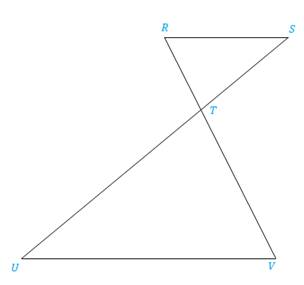Chapter 5.3, Problem 23E### Elementary Geometry for College St...

6th Edition
Daniel C. Alexander + 1 other
ISBN: 9781285195698

#### Solutions

Chapter
Section### Elementary Geometry for College St...

6th Edition
Daniel C. Alexander + 1 other
ISBN: 9781285195698
Textbook Problem
4 views

# In Exercises 17 to 24, complete each proof.Given: R S ¯ ∥ U V ¯ Prove: R T V T = R S V UPROOF Statements Reasons 1. ?2. ∠ R ≅ ∠ V and ∠ S ≅ ∠ U 3. ?4. ? 1. ?2. ?3. AA4. ?

To determine

To prove:

The statement RTVT=RSVU if the sides RS and UV are parallel, RS¯UV¯.

Explanation

Definition:

AA:

If two angles of one triangle are congruent to two angles of another triangle, then the triangles are similar.

CSSTP:

Corresponding sides of similar triangles are proportional.

Description:

Given that RS¯UV¯.

The given figure is shown below.

Figure

From the given figure, it is observed that the sides RS and UV are parallel, RS¯UV¯.

If two parallel lines are cut by a transversal, then the alternate interior angles are congruent.

Therefore, RV and SU.

The above mentioned AA definition, the two triangles RST and TUV are similar since the two angles of one triangle are congruent to two angles of another triangle. Hence, ΔRSTΔTUV

From the definition of CSSTP, corresponding sides of similar triangles are proportional

### Still sussing out bartleby?

Check out a sample textbook solution.

See a sample solution

#### The Solution to Your Study Problems

Bartleby provides explanations to thousands of textbook problems written by our experts, many with advanced degrees!

Get Started

#### Find more solutions based on key concepts